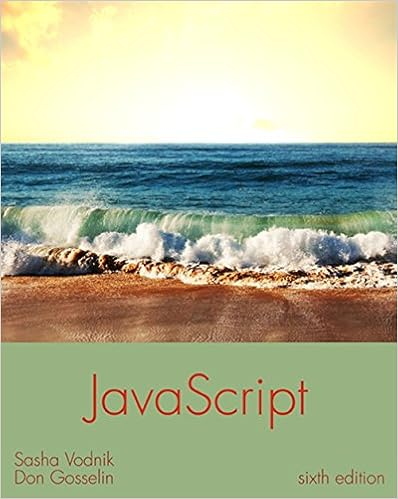# B modify the program in exercise 5a so that the two

• Homework Help
• 46
• 97% (175) 169 out of 175 people found this document helpful

This preview shows page 14 - 19 out of 46 pages.

##### We have textbook solutions for you!
The document you are viewing contains questions related to this textbook.The document you are viewing contains questions related to this textbook.
Chapter 2 / Exercise 4
JavaScript: The Web Warrior Series
VodnikExpert Verified
b. Modify the program in Exercise 5a so that the two entered values are passed to a method named getChoice(). The getChoice()method asks the user whether addition or subtraction should be performed and then passes the two values to the appropriate method, where the result is displayed.
##### We have textbook solutions for you!
The document you are viewing contains questions related to this textbook.The document you are viewing contains questions related to this textbook.
Chapter 2 / Exercise 4
JavaScript: The Web Warrior Series
VodnikExpert Verified
Programming Logic and Design, 8eSolutions 9-Pseudocode:startDeclarationsnum number1num number2output “Please enter two numbers”input number1, number2getChoice(number1, number2)stopvoid getChoice(num num1, num num2)Declarationsnum choiceoutput “Enter 1 for addition or 2 for subtraction: ”input choiceif choice = 1 thensum(num1, num2)15
Programming Logic and Design, 8e
6.Create the logic for a program that continuously prompts a user for a numeric value until the user enters 0. The application passes the value in turn to the following methods:
Programming Logic and Design, 8eSolutions 9-Pseudocode:startDeclarationsnum numbernum sumnum productnum QUIT = 0output “Enter a number or ”, QUIT, “ to quit”input numberwhile <> QUITdisplayNumbers(number)sum = calcSum(number)product = calcProduct(number)output “The sum is ”, sum, “ and the product is ”, productoutput “Enter a number or ”, QUIT, “ to quit”input numberendwhilestopvoid displayNumbers(num number)17
Programming Logic and Design, 8e
7.Create the logic for a program that calls a method that computes the final price for a sales transaction. The program contains variables that hold the price of an item, the salesperson’s commission expressed as a percentage, and the customer discount expressed as a percentage. Create a calculatePrice()method that determines the final price and returns the value to the calling method. The
•••